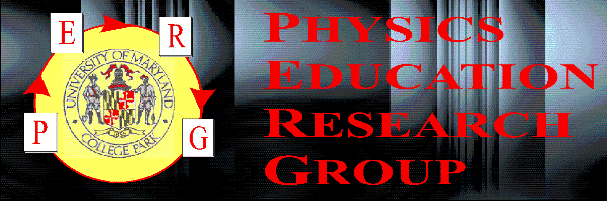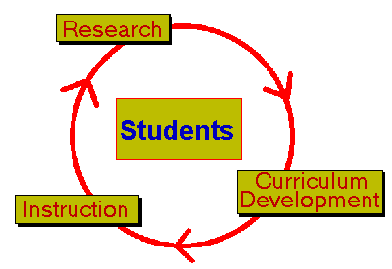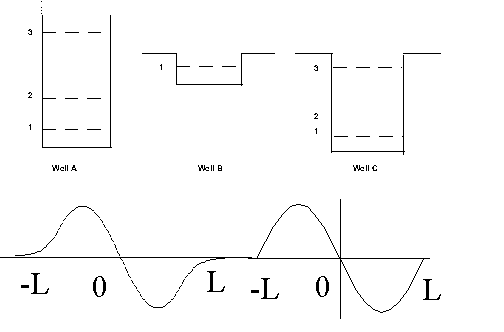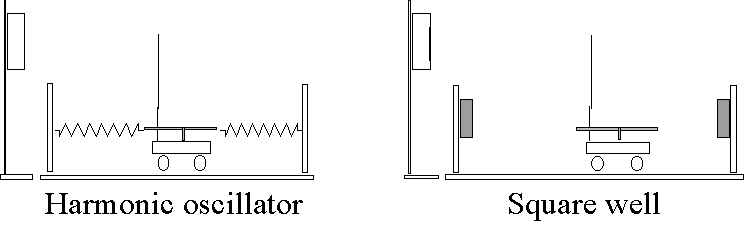Student Difficulties with Quantum Mechanics

Lei Bao, Pratibha Jolly, and Edward F. Redish
Physics Education Research Group
University of Maryland

Motivations

À Quantum Mechanics is an important yet difficult subject for students.

À Students often fail to achieve an understanding of fundamental concepts in the traditional teaching environment.

À If we want to get to quantum mechanics earlier and get students to learn it better we have to understand where the sticking points are.

Context for Investigation

Calculus-based physics course

À three 50-minute lectures per week

À one 50-minute group-learning tutorial

À one 2-hour lab

During Fall 94 and Spring 96 we taught 4 to 5 weeks of quantum mechanics at the end of the course.

Iterative process of research, instruction, and curriculum developmentÀ conducting investigation of student understanding

À applying results to the development of instructional strategies

À designing, testing, modifying and revising curriculum in a continuous cycle

Methods of investigation

À conceptual pretests / post tests

À observations of students in tutorials and office hours

À individual problem solving interviews

À homework / exams

Outline of our research

À For the Fall 94 class, we tried out three tutorials.

À During the instruction and tutorial, we made some preliminary observations of student difficulties in understanding quantum mechanics.

À A new tutorial was developed based on the previous results and was used in teaching (Spring 96).

À More detailed investigations were made during and after this tutorial.

Expectations and reality

Traditionally, when QM is taught, we assume students have:

À some understanding of basic probability

À the ability to read potential energy diagrams and link them with real physical systems

But according to our observations:

À most of the students don't have any previous knowledge about probability

À students have difficulties interpreting potential energy diagrams and often make incorrect links to   physical systems

Question:a. The wave functions shown are associated with two of the energy levels. Which ones do you think they are associated with? Explain your reasoning.

b. For these two states, approximately where in the well would the electron most likely be found? Explain your reasoning.

Correct answer for part b:  in both cases, the electrons will most likely be found near x = ▒ L/2.

Students responses:

À Less than 2% of the students gave the correct answer

À 40% leave it blank

À 36% use energy levels to describe the position

À 22% responded with other unrelated issues

We also find:

À a large number of students, 89%, failed to explain any reasoning

À 91% of the students didn't use x to describe position

Students have a tendency to interpret the 1-D potential well as a 2-D gravitational well.

" The electron will most likely be found at level 3."

" It will be most likely at bottom of the well."

" It will most likely be in the dashed lines."

And in a discussion later on same issues, one student said:

" It takes time to get out of the well because it has to climb up the wall. "

A new tutorial on classical probability and potential well

For the Spring 96, a new MBL tutorial was developed to address the conceptual difficulties in interpreting potential well diagrams and understanding probability.

The tutorial is intended to help students to:

À make a bridge to link the potential energy diagram with a physical system.

À create a "mental model" of a probability process. À -- the random picture method

The tutorial setup*À construct classical potential well with classical system

À study classical probability with random picture method

À practice in constructing diagrams and distribution

* This tutorial is based on a similar experiment in VQM developed by D. Zollman at Kansas State University.

The results from the conceptual post-test this year show:

À some improvements on the understanding of the classical issues

À more students use better reasoning in interpreting probability

À make better connections with velocity and energy

À students are more engaged into the relevant context and have better image of the real physical system.

The student performance on the final exam also show large percentage, 65%, can make correct connections with energy diagram to classical probability, and 81% of them chose the correct answer for quantum probability.

The interviews with students successful on these questions show continuing difficulties

À Inability to understand negative total energy

À Induced confusion in classical situation by misinterpreting quantum mechanical concepts

À Inability to understand the discontinuous potential energy diagrams